Olympiad Test: Embedded Figure - 2

# Olympiad Test: Embedded Figure - 2

Test Description

## 20 Questions MCQ Test Mathematical Olympiad Class 8 | Olympiad Test: Embedded Figure - 2

Olympiad Test: Embedded Figure - 2 for Class 8 2023 is part of Mathematical Olympiad Class 8 preparation. The Olympiad Test: Embedded Figure - 2 questions and answers have been prepared according to the Class 8 exam syllabus.The Olympiad Test: Embedded Figure - 2 MCQs are made for Class 8 2023 Exam. Find important definitions, questions, notes, meanings, examples, exercises, MCQs and online tests for Olympiad Test: Embedded Figure - 2 below.
 1 Crore+ students have signed up on EduRev. Have you?
Olympiad Test: Embedded Figure - 2 - Question 1

### Direction: In each of the following questions, fig. (X) is embedded in any one of the four alterative figures (a), (b), (c) or (d). Find the alternative which contains fig. (X) as its part.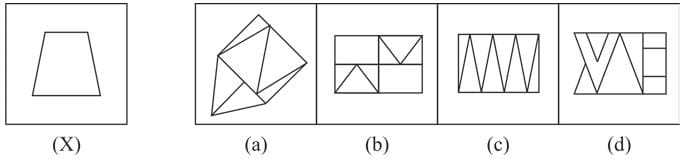Olympiad Test: Embedded Figure - 2 - Question 2

### Direction: In each of the following questions, fig. (X) is embedded in any one of the four alterative figures (a), (b), (c) or (d). Find the alternative which contains fig. (X) as its part.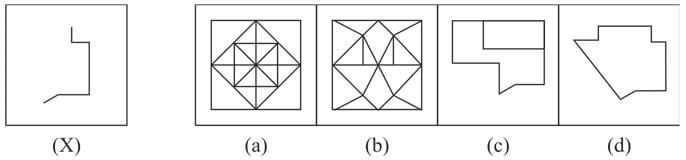Olympiad Test: Embedded Figure - 2 - Question 3

### Direction: In each of the following questions, fig. (X) is embedded in any one of the four alterative figures (a), (b), (c) or (d). Find the alternative which contains fig. (X) as its part.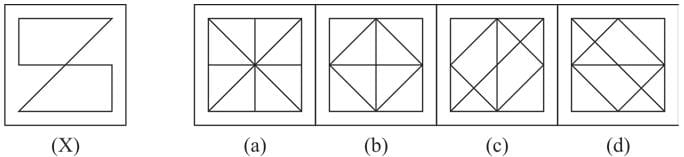Olympiad Test: Embedded Figure - 2 - Question 4

Direction: In each of the following questions, fig. (X) is embedded in any one of the four alterative figures (a), (b), (c) or (d). Find the alternative which contains fig. (X) as its part.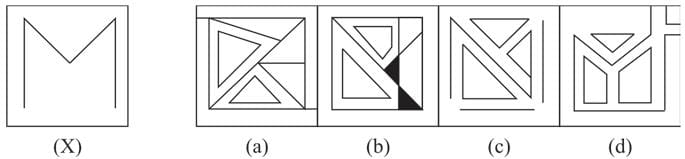Olympiad Test: Embedded Figure - 2 - Question 5

Direction: In each of the following questions, fig. (X) is embedded in any one of the four alterative figures (a), (b), (c) or (d). Find the alternative which contains fig. (X) as its part.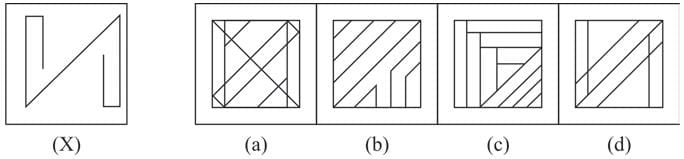Olympiad Test: Embedded Figure - 2 - Question 6

Direction: In each of the following questions, fig. (X) is embedded in any one of the four alterative figures (a), (b), (c) or (d). Find the alternative which contains fig. (X) as its part.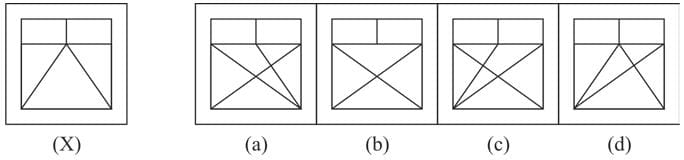Olympiad Test: Embedded Figure - 2 - Question 7

Direction: In each of the following questions, fig. (X) is embedded in any one of the four alterative figures (a), (b), (c) or (d). Find the alternative which contains fig. (X) as its part.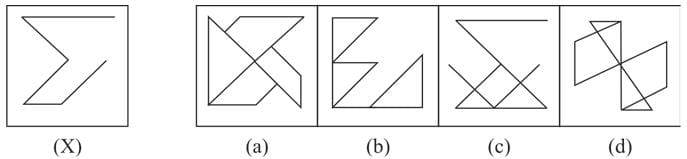Olympiad Test: Embedded Figure - 2 - Question 8

Direction: In each of the following questions, fig. (X) is embedded in any one of the four alterative figures (a), (b), (c) or (d). Find the alternative which contains fig. (X) as its part.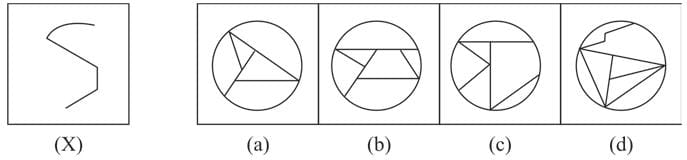Olympiad Test: Embedded Figure - 2 - Question 9

Direction: In each of the following questions, fig. (X) is embedded in any one of the four alterative figures (a), (b), (c) or (d). Find the alternative which contains fig. (X) as its part.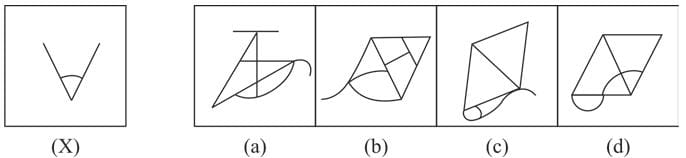Olympiad Test: Embedded Figure - 2 - Question 10

Direction: In each of the following questions, fig. (X) is embedded in any one of the four alterative figures (a), (b), (c) or (d). Find the alternative which contains fig. (X) as its part.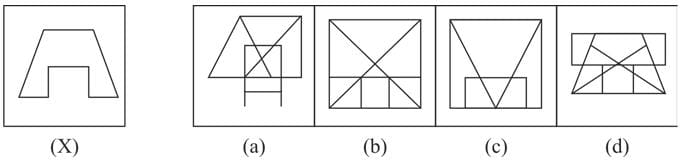Olympiad Test: Embedded Figure - 2 - Question 11

Direction: In each of the following questions, fig. (X) is embedded in any one of the four alterative figures (a), (b), (c) or (d). Find the alternative which contains fig. (X) as its part.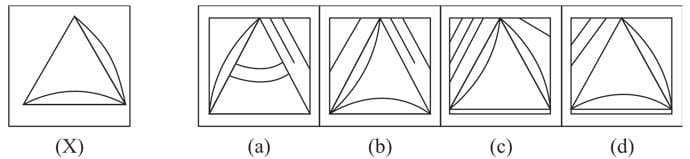Olympiad Test: Embedded Figure - 2 - Question 12

Direction: In each of the following questions, fig. (X) is embedded in any one of the four alterative figures (a), (b), (c) or (d). Find the alternative which contains fig. (X) as its part.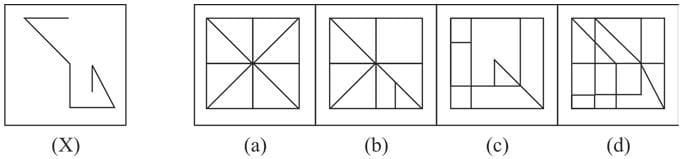Olympiad Test: Embedded Figure - 2 - Question 13

Direction: In each of the following questions, fig. (X) is embedded in any one of the four alterative figures (a), (b), (c) or (d). Find the alternative which contains fig. (X) as its part.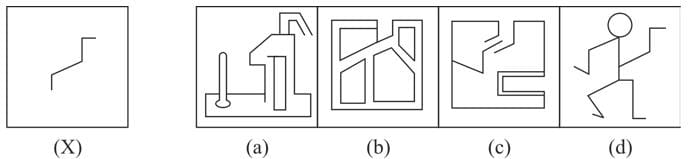Olympiad Test: Embedded Figure - 2 - Question 14

Direction: In each of the following questions, fig. (X) is embedded in any one of the four alterative figures (a), (b), (c) or (d). Find the alternative which contains fig. (X) as its part.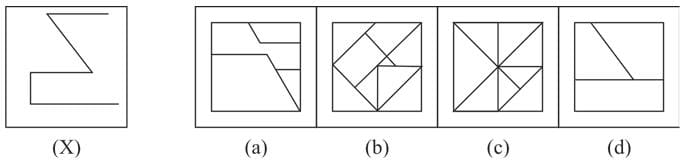Olympiad Test: Embedded Figure - 2 - Question 15

Direction: In each of the following questions, fig. (X) is embedded in any one of the four alterative figures (a), (b), (c) or (d). Find the alternative which contains fig. (X) as its part.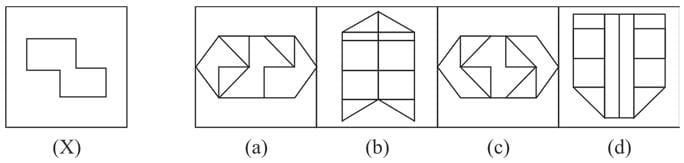Olympiad Test: Embedded Figure - 2 - Question 16

Direction: In each of the following questions, fig. (X) is embedded in any one of the four alterative figures (a), (b), (c) or (d). Find the alternative which contains fig. (X) as its part.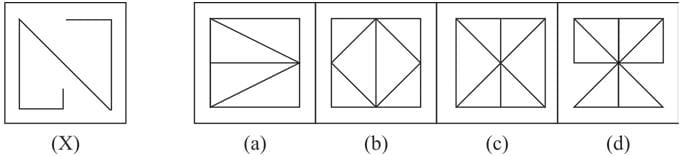Olympiad Test: Embedded Figure - 2 - Question 17

Direction: In each of the following questions, fig. (X) is embedded in any one of the four alterative figures (a), (b), (c) or (d). Find the alternative which contains fig. (X) as its part.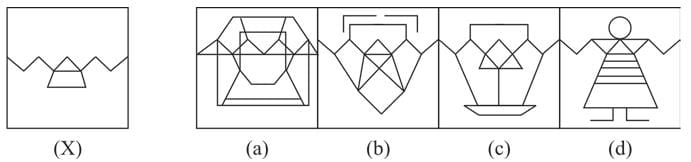Olympiad Test: Embedded Figure - 2 - Question 18

Direction: In each of the following questions, fig. (X) is embedded in any one of the four alterative figures (a), (b), (c) or (d). Find the alternative which contains fig. (X) as its part.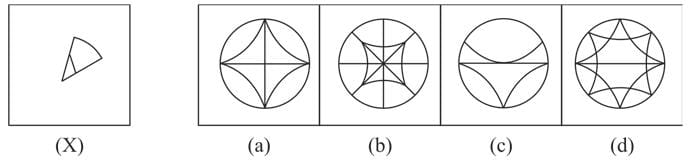Olympiad Test: Embedded Figure - 2 - Question 19

Direction: In each of the following questions, fig. (X) is embedded in any one of the four alterative figures (a), (b), (c) or (d). Find the alternative which contains fig. (X) as its part.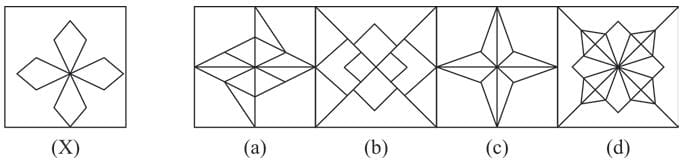Olympiad Test: Embedded Figure - 2 - Question 20

Direction: In each of the following questions, fig. (X) is embedded in any one of the four alterative figures (a), (b), (c) or (d). Find the alternative which contains fig. (X) as its part.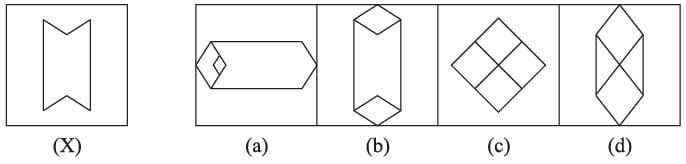(Scan QR code)